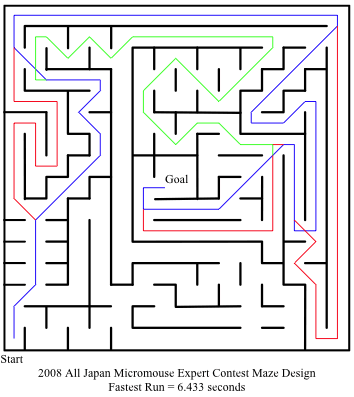by Alan Dibley

The translation of a route from one using 90 degree turns only to one using a combination of 45, 90 and 135 degree turns is not an obvious or trivial task. There are several possible approaches. this method recognises that there are only three kinds of action in an orthogonal route. Thus it seems to make sense to represent those values as a base three, or ternary, number. A simple translation process can then take these values as triplets and look up a translated move in a table.

Starting with the maze used in the 2008 expert finals in Japan:I used the blue route shown in David Otten’s report on the Japan ’08 event which is described in the following sequence of 90 degree moves:

FFRLFFFRLRLRLFLRRLLFFRFRRLLRRLLRFFFFFFRRLRLRRLRLLRLFLRLLRFRLFRRLRLRFFFRRF

which converts to this sequence of 45 and 90 degree moves:

FFrflFFrfffffllfRfxFFRFRrfLfRfLfrFFFFFRrffffRfffLffllffLfrrflRrffffrFFRRF

using the notation:-
F = forward orthogonally one cell
L/R = turn left or right through 90 degrees
l/r = turn left or right through 45 degrees
f = forward at 45 degrees
x/z = turn left or right through 135 degrees

Logically the moves start and end at the centre points of the sides of squares, and turns are associated with the square at entry. So the first ‘l’ in the sequence actually takes place in square 0xD0 in my notation, with square 0x00 at top left and 0xFF at bottom right. Thezeus moves always start before the mouse has actually arrived in the square, so this works OK if the 45 degree turns are a little more abrupt than I would like. My logic treats every move as starting before the mouse (the centre of the axle in a wheel-chair machine) has left the preceding square. The wall-sensors are already in the subject square. There is variation between 45 and 90 moves in the length of short straight rmotion before turning starts to allow some speed adjustment.

‘l’ and ‘r’ moves start with a short(-ish) curve before a long(-ish) straight, to allow this method to work – the straight ends before the next square is actually entered. For 45-to-90 l’s and r’s this straight is most of the square width. Thus the first move in that sequence of three apparent ‘F’ moves (in 0xC1) is actually the tail end of an ‘l’ move.

To translate from the ’90’ version to the ’45’ version attach the values 0,1,and 2 to the moves F, L and R, thus:-

FFRLFFFRLRLRLFLRRLLFFRFRRLLRRLLRFFFFFFRRLRLRRLRLLRLFLRLLRFRLFRRLRLRFFF….
0021000212121012211002022112211200000022121221211010121120210221212000….
*

The first move is always an F. For the rest of the moves take the triplet of digits associated with the preceding move, this move, and the next move, and interpret them as a ternary number. (A number to base 3 uses 9, 3 and 1 in place of 100, 10 and 1 in decimal numbers.) Thus the value of the ternary number associated with the first L move marked with an asterisk is 210(base 3) = 18 + 3 + 0 = 21(base 10). Using this information (and some mind-numbingly tedious calculations of lots of ternary numbers) we can generate translation tables to allow easy fast conversion – one column for use when the mouse is travelling orthogonally and one for use when travelling diagonally. Blanks represent illegal combinations.

 Orthogonal route New diagonal route Moves Ternary Decimal Orthogonal Diagonal F F F 000 00 F F F L 001 01 F F F R 002 02 F F L F 010 03 L F L L 011 04 L F L R 012 05 l F R F 020 06 R F R L 021 07 r F R R 022 08 R L F F 100 09 F l L F L 101 10 F l L F R 102 11 F l L L F 110 12 l x L L L 111 13 – L L R 112 14 F L L R F 120 15 f L R L 121 16 F f L R R 122 17 f R F F 200 18 R r R F L 201 19 r R F R 202 20 r R L F 210 21 f R L L 211 22 f R L R 212 23 f R R F 220 24 z R R L 221 25 R R R R 222 26

Switch between columns on the condition of a flag ‘f45’ which is toggled at every x, l, r and z move.

Note that many values are invalid, for instance LLL and RRR are impossible combinations. Some values are missing because they would not normally occur. For example, the sequence LRF (15) does not have an entry in the orthogonal column because it should only appear when the mouse is at the end of a diagonal run. Also, there may be be move combinations not occurring in the Japanese maze, which means that the method must be tested before use in competition. The tables don’t look ‘proper’ somehow???

The data can conveniently be held in two strings of characters or a two-dimensional array of characters.

There are other ways of achieving the result by similar numerical methods, but this one seems efficient in the environment of a small processor.

Comments, corrections and suggestions for improvement welcome.

[The above was presented by Alan Dibley at the 2009 Minos Conference held at Royal Holloway College]

### This Post Has One Comment

1. Alan Dibley

This is only an outline method. I assumed it would be easy and productive for other folk to work out details for themselves. For instance there are more types of move to be catered for, 14 in all. Note that all moves which change from perpendicular motion to diagonal motion are quite different to those from diagonal to perpendicular. If you work it out for yourself it becomes clear(???).

This site uses Akismet to reduce spam. Learn how your comment data is processed.